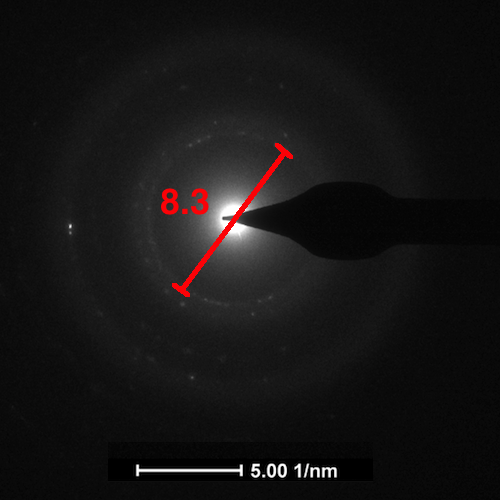# SAED d value and XRD Peak Position calculator

## SAED d value and XRD Peak Position calculator

Absorption Peak Wavelength (nm)

Results
(nm) d value
(2θ) XRD Peak Position

#### Calculation Tutorial:

STEP1: Take the raw image of Selected Area Electron Diffraction (SAED) from Transmission Electron Microscope (TEM)STEP2: Now enter the distance (nm) between two bright spots into "Distance between 2 bright spots" column of the calculator. You should get the calculated result of d value in the field below. SAED d value calculation

NOTE: XRD peak position is calculated by taking Laser wavelength as 0.15418 and n value as 1. The distance is in reciprocal space. We have made the calculator such that if you measure the distance directly from 1/nm scale (as shown in image) it would give you the exact d value results.

Theory behind d value calculation: Selected Area Electron Diffraction (SAED) is very important technique to determine the crystal structure of any material. It is a complementary technique in Transmission Electron Microscope (TEM), in which the Electrons are diffracted at a selected area and bright spots with dark background are observed as a result of this.
Distance between two bright spots (D) = 2 × Radius of that circle
The distance is in reciprocal space so we take inverse to convert it into real space
Thus, the formula for Interplanar spacing (d):
Interplanar spacing (d) = 2 / Distance between two bright spots

1.It’s difficult to locate well informed individuals
on this subject, but you sound like you understand what you’re talking about!
Thanks

2.How to calculate the d-spacing of single crystals using SAED pattern

3.How did you calculate d without camera constant??? This doesn't look correct.

4.This does not take into account camera constant or single crystal patterns. This only works if measuring the diameter of a circle produced by polycrystalline material.